# Auto stopping distance

Under optimum conditions on a dry road, the coefficient of friction may be as high as 0.8, so that you could decelerate at 0.8g = 0.8 x 9.8 m/s^2 = 7.8 m/s^2.
a. If you are traveling at 55 mi/h (24.6 m/s), how long will it take you to stop?
b. How far will you travel while stopping?

Solution:

All constant acceleration problems can be solved using the basic motion equations.

 a. Using the basic motion equation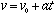, or solving for t,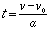In the equation, v is the velocity at a chosen time t. In this problem the initial velocity is given, and you know that v=0 since it is the velocity at the time when you stop.

Examining the known and unknown quantities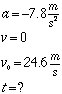leads to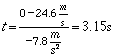b. The stopping distance may be calculated from the motion equation: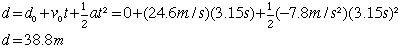Alternatively, the distance may be calculated from the combined motion equation form which eliminates the time from the calculation.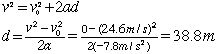Auto stopping distance discussion Example problems in mechanics
Index

Motion with constant acceleration
HyperPhysics***** Mechanics Go Back